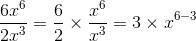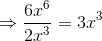ISEE Upper Level Math : Numbers and Operations

Example Questions

Example Question #15 : How To Multiply Exponents

What is the value of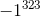?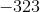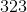Explanation:

1 raised to any exponent will always be 1.

-1 will be equal to 1 when the exponent is even and will be equal to -1 when the exponent is odd.

Given that 323 is odd,is equal to -1.

Example Question #16 : How To Multiply Exponents

Simplify the following: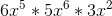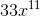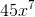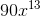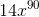Explanation:

Simplify the following:Let's begin by recalling two rules

1) When multiplying variables with a common base, add the exponents.

2) When multiplying variables with a common base, multiply the coefficients.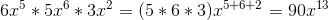Example Question #1 : How To Divide Exponents

Simplify: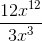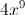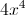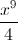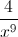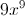Explanation:

Separate the fraction and apply the quotient of powers rule: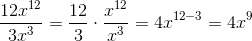Example Question #2 : How To Divide Exponents

Simplify: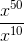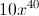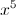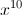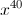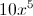Explanation:

Apply the quotient of powers rule.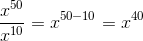Example Question #81 : Numbers And Operations

Evaluate: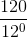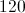The expression is undefined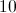Explanation:

Any nonzero number raised to the power of 0 is equal to 1, so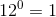, and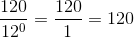Example Question #4 : How To Divide Exponents

Evaluate: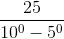The expression is undefined.The expression is undefined.

Explanation:

Any nonzero number raised to the power of 0 is equal to 1, so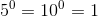. Therefore,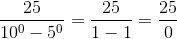However, an expression with a denominator of 0 is undefined, so that is the correct choice.

Example Question #5 : How To Divide Exponents

Simplify: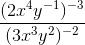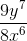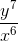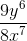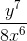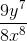Explanation:

Based on the negative exponent rule we have: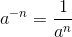Which says negative exponents in the numerator get moved to the denominator and become positive exponents. And negative exponents in the denominator get moved to the numerator and become positive exponents. So we can write: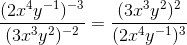Based on the power rule for exponents we can write: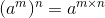That means; to raise a power to a power we need to multiply the exponents.So we have: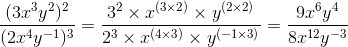Separate the equation: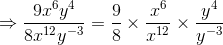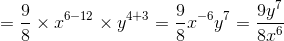Example Question #6 : How To Divide Exponents

Simplify: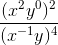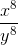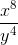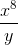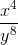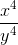Explanation:

Based on the zero-exponent rule we have: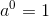That means any non-zero number raised to the zero power is equal to. So we can write: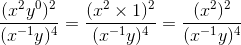Based on the power rule for exponents we can write:That means; to raise a power to a power we need to multiply the exponents. In addition based on the negative exponent rule we have:Which says negative exponents in the numerator get moved to the denominator and become positive exponents. And negative exponents in the denominator get moved to the numerator and become positive exponents. So we can write: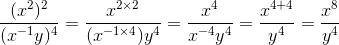Example Question #7 : How To Divide Exponents

Evaluate: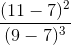Explanation:

First we need to subtract the numbers in parentheses. So we can write: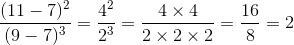We can alternatively write: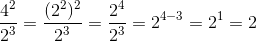Example Question #8 : How To Divide Exponents

Simplify: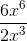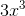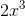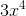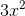Explanation:

Based on the quotient rule for exponents, we know that for any non-zero numberand any integersand,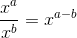.

We should separate the fraction and apply the quotient rule for exponents: